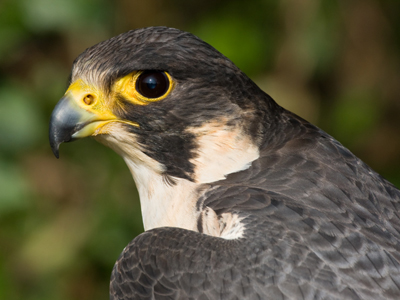A peregrine falcon can fly at 90 km/h but can dive on its prey at much greater speeds.

# Speed 02

## Revise what you know about the speed at which things travel. What is the fastest bird?

### Scroll down to play the quiz

See how much you know about speed in our second KS3 Science quiz on the subject. The fastest land animal is the cheetah which has been recorded running at a speed of between 110 and 120 km/h. The fastest bird is the peregrine falcon which has been recorded diving to catch its prey at an astonishing 389 km/h.

That is a bit of a cheat though because it is not really flying, it is simply falling from a great height, but is under control. Human skydivers in free fall (the part of the skydive before the parachute is opened) can achieve speeds of almost 200 km/h, half that of the peregrine.

In 2012, Felix Baumgartner ascended to a height of 39 km altitude to do a free fall parachute jump. The atmosphere is very thin at that height and he was able to fall much faster than someone in free fall from 10 km or lower.

He reached a speed of 1,357 km/h which was faster than the speed of sound. Back on Earth, the fastest humans can run is about 40 km/h, which is not even fast enough to beat a domestic cat in a race (they can sprint at 45 - 50 km/h when they need to).

One of the key skills you will learn in science is how to rearrange an equation to make it calculate what you want. So from the basic equation of speed equals distance divided by time, you can work out the distance travelled if you multiply the speed by the time the object was moving at that speed.

1.
If time is measured in hours, speed in mph, distance .......
is in centimetres
is in metres
is in kilometres
is in miles
Professional scientists would never use mph as a measure for speed in their experiments
2.
A cyclist has a speed of 10 km/h. She cycles for 2 hours - how far does she go?
5 kilometres
12 kilometres
20 kilometres
25 kilometres
Distance = 10 km/h x 2 h = 20 kilometres
3.
An athlete runs at 8 m/s for 1 minute. How far do they go?
48m
80m
480m
4.8km
1 minute = 60 seconds, so distance = 8 m/s x 60 s = 480 m
4.
If time is measured in hours, speed in km/h, distance .......
is in centimetres
is in metres
is in kilometres
is in miles
You don't always need to express distance in metres per second, in fact it can sometimes be more helpful to know speeds in more familiar everyday units like km/h
5.
A cyclist has a speed of 10 km/h. She cycles for 120 minutes - how far does she go?
10 kilometres
12 kilometres
20 kilometres
1,200 kilometres
120 minutes = 2 hours, so distance = 10 km/h x 2 h = 20 km
6.
If a peregrine falcon flies at 90 km/h, how far could it fly in 20 minutes?
4.5 kilometres
18 kilometres
30 kilometres
1,800 kilometres
20 minutes = 20/60 = 1/3 hour, so distance = 1/3 h x 90 km/h = 30 km
7.
If time is measured in seconds, speed in m/s, distance .......
is in centimetres
is in metres
is in kilometres
is in miles
This is the basic SI unit of distance
8.
A dog runs at 15 m/s for 30 seconds. How far does it travel?
45m
450m
2km
4.5km
15 m/s x 30 s = 450 m
9.
A car travels at 40 mph for 60 minutes. How far does it go?
40 kilometres
40 miles
60 miles
240 miles
60 minutes = 1 hour, so distance = 40 mph x 1 h = 40 miles
10.
A car travels at 50 mph for 2 hours. How far does it go?
10 miles
50 miles
100 miles
100 kilometres
Distance = 50 mph x 2 h = 100 miles